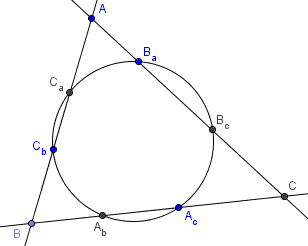# Carnot's Theorem for Conics

The following theorem is due to Lazare Nicolas Marquerite (count) Carnot (13 May 1753 - 2 August 1823):

Assume a conic meets the side lines of $\Delta ABC$ in points $A_a,A_b$ (on $BC$), $B_a,B_c$ (on $AC$), and $C_a,C_b$ (on $AB),$ in the order implied in the diagram below:Then

$AC_a\cdot AC_b\cdot BA_b\cdot BA_c\cdot CB_c\cdot CB_a=\\ \space\space AB_a\cdot AB_c\cdot CA_c\cdot CA_b\cdot BC_b\cdot BC_a,$

where all the segments are signed.

### Proof

I shall prove that first for circles and then argue that the property is in fact projective and, therefore, holds for all non-degenerate conics.

By the Power of a point theorem, $AC_a\cdot AC_b=AB_a\cdot AB_c,$ $BA_b\cdot BA_c=BC_b\cdot BC_a,$ and $CB_c\cdot CB_a=CA_c\cdot CA_b.$ The product of the three is exactly Carnot's identity.

This proves Carnot's theorem for circles. Now let's rewrite the identity differently:

$\displaystyle \big(\frac{AC_a}{BC_a}\cdot\frac{AC_b}{BC_b}\big)\cdot\big(\frac{BA_b}{CA_b}\cdot\frac{BA_c}{CA_c}\big)\cdot\big(\frac{CB_c}{AB_c}\cdot\frac{CB_a}{AB_a}\big)=1.$

The above expression is of the sort that remain invariant under projective mappings, implying that Carnot's theorem remains valid for all non-degenerate conic sections.

As an example, the six points of tangency of the excircles with the extensions of the sides of a triangle lie on a conic and so do the points of tangency of the incircle and the excircles on the sides proper.

I am grateful to Emmanuel Antonio José García for drawing my attention to Carnot's theorem and to Hubert Shutrick for supplying an alternative proof.